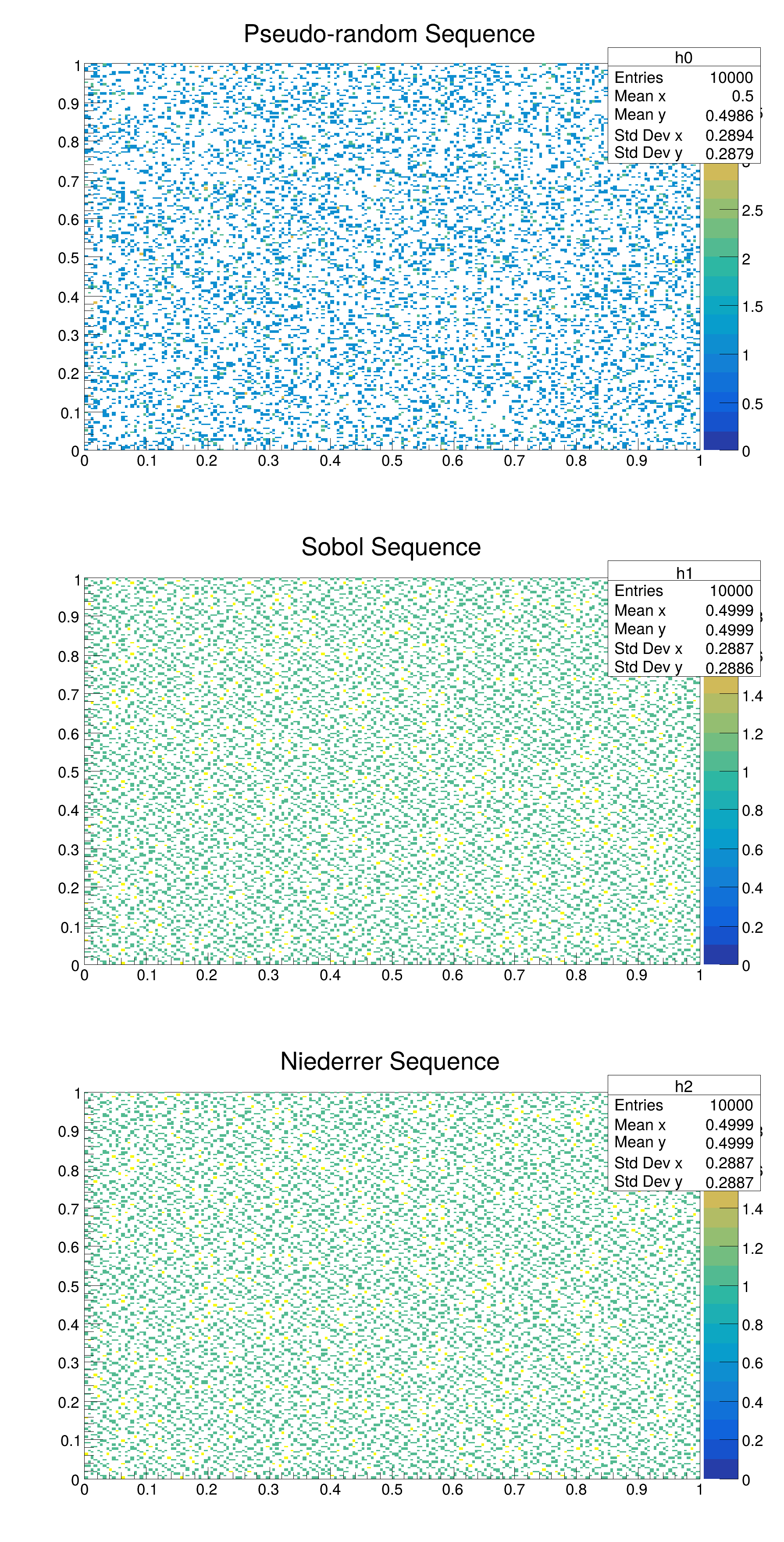ROOT   Reference Guide
Searching...
No Matches
quasirandom.C File Reference

## Detailed DescriptionExample of generating quasi-random numbersTime for gRandom Real time 0:00:00, CP time 0.000
Time for Sobol Real time 0:00:00, CP time 0.000
Time for Niederreiter Real time 0:00:00, CP time 0.000
number of empty bins for pseudo-random = 31139
number of empty bins for sobol = 30512
number of empty bins for niederreiter-base-2 = 30512
(int) 0
#include "Math/Random.h"
#include "TH2.h"
#include "TCanvas.h"
#include "TStopwatch.h"
#include <iostream>
using namespace ROOT::Math;
int quasirandom(int n = 10000, int skip = 0) {
TH2D * h0 = new TH2D("h0","Pseudo-random Sequence",200,0,1,200,0,1);
TH2D * h1 = new TH2D("h1","Sobol Sequence",200,0,1,200,0,1);
TH2D * h2 = new TH2D("h2","Niederrer Sequence",200,0,1,200,0,1);
// quasi random numbers need to be created giving the dimension of the sequence
// in this case we generate 2-d sequence
// generate n random points
double x;
for (int i = 0; i < n; ++i) {
r0.RndmArray(2,x);
h0->Fill(x,x);
}
std::cout << "Time for gRandom ";
w.Print();
w.Start();
if( skip>0) r1.Skip(skip);
for (int i = 0; i < n; ++i) {
r1.Next(x);
h1->Fill(x,x);
}
std::cout << "Time for Sobol ";
w.Print();
w.Start();
if( skip>0) r2.Skip(skip);
for (int i = 0; i < n; ++i) {
r2.Next(x);
h2->Fill(x,x);
}
std::cout << "Time for Niederreiter ";
w.Print();
TCanvas * c1 = new TCanvas("c1","Random sequence",600,1200);
c1->Divide(1,3);
c1->cd(1);
h0->Draw("COLZ");
c1->cd(2);
// check uniformity
h1->Draw("COLZ");
c1->cd(3);
h2->Draw("COLZ");
// test number of empty bins
int nzerobins0 = 0;
int nzerobins1 = 0;
int nzerobins2 = 0;
for (int i = 1; i <= h1->GetNbinsX(); ++i) {
for (int j = 1; j <= h1->GetNbinsY(); ++j) {
if (h0->GetBinContent(i,j) == 0 ) nzerobins0++;
if (h1->GetBinContent(i,j) == 0 ) nzerobins1++;
if (h2->GetBinContent(i,j) == 0 ) nzerobins2++;
}
}
std::cout << "number of empty bins for pseudo-random = " << nzerobins0 << std::endl;
std::cout << "number of empty bins for " << r1.Name() << "\t= " << nzerobins1 << std::endl;
std::cout << "number of empty bins for " << r2.Name() << "\t= " << nzerobins2 << std::endl;
int iret = 0;
if (nzerobins1 >= nzerobins0 ) iret += 1;
if (nzerobins2 >= nzerobins0 ) iret += 2;
return iret;
}
User class for MathMore random numbers template on the Engine type.
Definition QuasiRandom.h:60
Documentation for the Random class.
Definition Random.h:43
void RndmArray(int n, double *array)
Generate an array of random numbers between ]0,1] 0 is excluded and 1 is included Function to preserv...
Definition Random.h:71
The Canvas class.
Definition TCanvas.h:23
virtual Int_t GetNbinsY() const
Definition TH1.h:297
virtual Int_t GetNbinsX() const
Definition TH1.h:296
virtual Int_t Fill(Double_t x)
Increment bin with abscissa X by 1.
Definition TH1.cxx:3325
void Draw(Option_t *option="") override
Draw this histogram with options.
Definition TH1.cxx:3047
virtual Double_t GetBinContent(Int_t bin) const
Return content of bin number bin.
Definition TH1.cxx:5010
2-D histogram with a double per channel (see TH1 documentation)}
Definition TH2.h:301
Double_t GetBinContent(Int_t binx, Int_t biny) const override
Definition TH2.h:89
Int_t Fill(Double_t) override
Invalid Fill method.
Definition TH2.cxx:347
Stopwatch class.
Definition TStopwatch.h:28
void Start(Bool_t reset=kTRUE)
Start the stopwatch.
return c1
Definition legend1.C:41
Double_t x[n]
Definition legend1.C:17
const Int_t n
Definition legend1.C:16
TH1F * h1
Definition legend1.C:5

Definition in file quasirandom.C.